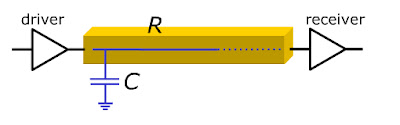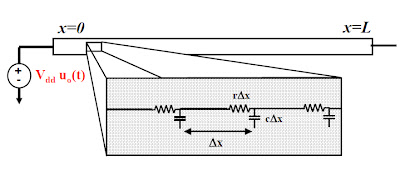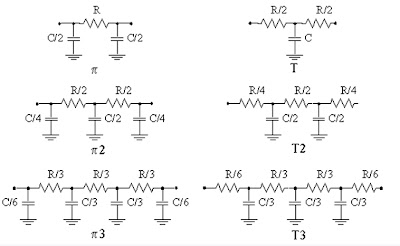## Index

 STA & SI Chapter1 Chapter2 Chapter3 Chapter4 Chapter5 Chapter6 Chapter7 Chapter8 Introduction Static Timing Analysis Clock Advance STA Signal Integrity EDA Tools Timing Models Other Topics

 Extraction & DFM Chapter1 Chapter2 Chapter3 Chapter4 Chapter5 Chapter6 Introduction Parasitic Interconnect Corner (RC Corner) Manufacturing Effects and Their Modeling Dielectric Layer Process Variation Other Topic

## Sunday, September 11, 2011

### Delay - "Interconnect Delay Models" : Static Timing Analysis (STA) basic (Part 4b)

 STA & SI:: Chapter 2: Static Timing Analysis 2.1 2.2 2.3a 2.3b 2.3c 2.4a Timing Paths Time Borrowing Basic Concept Of Setup-Hold Basic Concept of Setup-Hold Violation Examples:S-H Time/Violation Timing Path Delay 2.4b 2.4c 2.5a 2.5b 2.6a 2.6b Interconnect Delay Models Delay - Wire Load Model Maximum Clock Frequency Calculate “Max Clock Freq”-Examples Fix Setup-Hold Violation-1 Fix Setup-Hold Violation-2 2.6c 2.7a 2.7b 2.7c 2.8 Fix Setup-Hold Violation-3 Incr/Decr Delay Method-1 Incr/Decr Delay Method-2 Incr/Decr Delay Method-3 10 ways to fix Setup-Hold Violation.

Static Timing analysis is divided into several parts:

In the previous post we have discussed about the way tool calculate the max and min delay in a circuit. Now we will discuss other basics of the Delay and delay calculation. During your day to day work (in Semiconductor Field) or say in different Books, you come across different terminology related to the delays. There is a long list of that.
• Input Delay
• Output Delay
• Cell Delay
• Net Delay
• Wire Delay
• Slope Delay
• Intrinsic Delay
• Transition Delay
• Connect Delay
• Interconnect Delay
• Propagation Delay
• Min/Max Delay
• Rising/Falling Delay
• Gate Delay
• Stage delay
Fortunately or say luckily out of the above mention long list few are just synonym of other and few are interrelated to each other . Like Net delay also know as Wire Delay , Interconnect delay. Broadly we can divide this Long List into 2 type of delay.  Net Delay (Wire delay) and Cell Delay.  ( Note : Stage Delay = Net delay + Cell Delay. )
So let’s discuss these one by one. In digital design, a wire connecting pins of standard cells and blocks is referred to as a NET. A net
• Has only one driver
• Has a number of fanout cells or blocks.
• Can travel on multiple metal layers of the chip.
“Net Delay” refers to the total time needed to charge or discharge all of the parasitic (Capacitance / Resistance / Inductance) of a given Net. So we can say that Net delay is a function of
• Net Resistance
• Net Capacitance
• Net Topology
Now to calculate the Net delay, the wires are modeled in different ways and there are different way to do the calculation. Practically, when you are applying a particular delay model in a design , then you have to apply that to all cells in a particular library. You cannot mix delay models within a single library. There are few recommendations provided by experts or say experienced designer regarding the application of a particular Delay model in a design and that depends on
• Technology of design.
• At what stage you are ? Or say at what stage you want to apply a delay model.
• How accurately you want to calculate the delay.
Note : Ideally Till the physical wire is not present in you design, you cannot calculate the Net delay. Reason is ... If wire is not present , you have no idea about the Length/Width of the wires. SO YOU CANN'T CALCULATE THE ACCURATE VALUES OF PARASITIC OR SAY DELAY VALUE OF THE WIRE. But here main point is  accurate value, means there is possibility of inaccurate or say approximate value of delay value before physical laying of wire in a design.

There are several delay models. Those which can provide more accurate result, takes more runtime to do the calculation and those which are fast provides less accurate value of delay. Lets discuss few of them. Most popular delay models are -
• Lumped Capacitor Model
• Lumped RC model
• Distributed RC model
• Pi RC network
• T RC network
• RLC model
• Elmore Delay model
• Transmission Line Model

Lumped Capacitor Model.
• Model assume that wire resistance is negligible.
• Source driver sees a single loading capacitance which is the sum of total capacitance of the interconnect and the total loading capacitance at the sink.
• In past (higher technology-350nm and so), capacitor was dominating and that’s the reason in the model we have only capacitance.
• Older technology had wide wires,
•  More cross section area implies less resistance and more capacitance.
• So Wire model only with capacitance.
•  In the Fig R=0Lumped Capacitor Model

Lumped RC (Resistance Capacitance) model:
• As the feature size decreases to the submicron dimensions, width of the wire reduced.
• Resistance of wire is no longer negligible.
• Have to incorporate the resistance in our model. And that’s the reason Lumped RC model (or say RC tree) comes into picture.

In lumped RC model the total resistance of each wire segment is lumped into one single R, combines the global capacitive into single capacitor C.Lumped RC Model

Distributed RC model:

Distributed means RC is distributed along the length of the wire. The total resistance (Rt) and capacitance (Ct) of a wire can be expressed as
Rt = Rp * L
Ct = Cp * L

Where
Cp and Rp are Capacitance and Resistance per unit length.
L is the length of the wire.

Ideally, distributing the resistance and capacitance of a wire in very small portion of the wire (say delta) give you the better performance. Now to find out the total capacitance and resistance we use the differential equation. Distributed RC model provides better accuracy over lumped RC model. But this type of model is not practically possible.Distributed RC Model

The distributed RC model can be configured by 2 ways based on the structure or say shape (pi and T). Following is the pictorial view.

T model:
• Ct is modeled as a half way of the resistive tree.
• Rt is broken into 2 sections (each being Rt/2 )

Pi Model:
• Ct is broken into 2 sections (each being Ct/2) are connected on either side of the resistance.
• Rt is in between the capacitances.

For practical purpose, wire-models with 5-10 elements/nodes are used to model the wire.  It will provide the more accurate result. For N element section

For T network:
• Intermediate section of resistance are equal to Rt/N.
• Intermediate section of Capacitance are modeled by Ct/N
• End section of Resistance are equal to Rt/(2N).
• This T Network is represented as TN model.

For Pi network:
• Intermediate section of resistance are equal to Rt/N.
• Intermediate section of Capacitance are modeled by Ct/N
• End section of Capacitance are equal to Ct/(2N).
• This Pi Network is represented as PiN model.2 Types of Distributed RC Model (Pi- Model and T- Model)

Note: Lumped Vs Distributed RC wire:

Following is the comparison between the Lumped and distributed RC network. It will help you to understand in terms of uses of the both type of network in terms of accuracy and runtime.

Following is the Step Response of Lumped Vs Distributed RC line.Step Response Of Lumped and Distributed RC Network

Below comparison Table will give you more accurate picture.

 Output Potential range Time Elapsed Distributed RC Network Lumped RC network 0 to 90% 1.0RC 2.3RC 10% to 90% (rise time) 0.9RC 2.2RC 0 to 63% 0.5RC 1.0RC 0 to 50% 0.4RC 0.7RC 0 to 10% 0.1RC 0.1RC

RLC model

In the past since the design frequency was low so the impedance (wL) was dominated by Resistance (wL << R). So we are not caring “L”. However if you are operating at higher frequency and use the wider wire that reduce the resistivity then we have to take account the inductance into our modeling.Distributed RLC Model

﻿

In next part we will discuss Wire Load Delay Model...